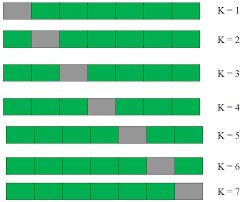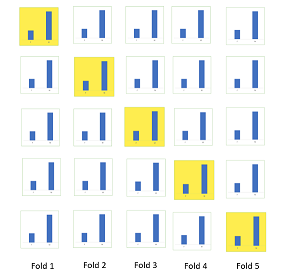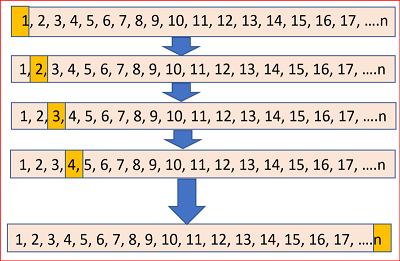gridsearch-eng

A typical machine learning process is training different models in a dataset and selecting the one with the best performance. There are several factors that can help determine the best performance of the algorithm. One such factor is the performance of the cross-validation set and another factor is the selection of parameters (hyper parameter tuning).

##### Cross-Validation¶

Data prepared for machine learning is usually divided into training and test set; the training set is then used to train the model and the test set is used to evaluate the model’s performance. However, this approach can lead to some problems. In other words, the accuracy obtained in one test is very different to the accuracy obtained in another test set using the same algorithm. The solution to this problem is to use K-Fold Cross-Validation to evaluate performance, where K is any number. We divide the data into K-chunks. Of these K-chunks, some sets (all but one, K-1) are used for training while the rest of the set is used for testing. The algorithm is trained and tested K times each time a new set is used as a test set while the remaining sets are used for training. Ultimately, the K-Fold Cross-Validation score is the average of the scores obtained on each set.

There are several cross-checking variances: K-Fold, Stratified K-Fold, Leave One Out, Repeated.

K-Fold
The routine has a single parameter k that corresponds to the number of groups into which a given data sample is to be divided. If k = 10, the data set will be divided into 10 equal parts -> 9 training sets and 1 test set, then modeling and so on 10 times (each test part is different) – and the result is the average of all models. If k = 1, it works like the already known function: train / test split. If we have 100 rows in our dataset and k = 5 then we have 100/5 = 20 rows in each subset (fold1, fold2, fold3, fold4 and fold5). Then we repeat it 5 times. For the first iteration, our test set is fold1 and the rest are training sets. Then we calculate the testing error (error1). The next time the test set will be fold2 and the rest are training sets, we get error2. We repeat this 5 times. Then the accuracy will be calculated:
Training accuracy = (error1 + …. + error5) / 5

from IPython.display import Image
Image(filename="img/kfold.png")Stratified K-Fold

Dividing the data into parts may be governed by criteria such as ensuring that each sample has the same proportion of observations with a specified categorical value, such as a class score value. This is called stratified cross-validation.

Image(filename="img/strat.png")Leave One Out

In this type of cross-checking, the number of subsets is equal to the number of cases we have in the dataset. Then we value ALL these subsets and build our model with the mean, the next step is to test the model against the last match. As we would get a large number of training sets (equal to the number of samples) this method is computationally very expensive and should be used for small data sets. If your dataset is large, it is most likely better to use another method such as kfold.

Image(filename="img/loov.png")Let’s see an example of how cross-checking works, we will start as always with importing the necessary libraries and some data:

import numpy as np
from sklearn.model_selection import cross_val_score
from sklearn.model_selection import KFold
from sklearn.neighbors import KNeighborsClassifier

data = load_iris()
X, y = data.data, data.target


We will use the basic version of kfold, with a bit more variance. We divide the data into 10 subsets, model it with the KNN algorithm and obtain the average accuracy of the model and standard deviation.

kfold = KFold(n_splits=10)
model_knn = KNeighborsClassifier()
result = cross_val_score(model_knn, X, y, cv=kfold)
print("KFold KNN: %.3f%% (%.3f%%)" % (result.mean()*100.0, result.std()*100.0))

KFold KNN: 93.333% (8.433%)


The result of the selected model accuracy is 93%, this is the maximum accuracy among these subsets. For comparison, we will use train / test split, the result is similar, but it must be remembered that this is the result of a single data division into test and training. The result with kfold is more realistic.

from sklearn.model_selection import train_test_split
X_train, X_test, y_train, y_test = train_test_split(X,y, test_size = 0.20)
model_knn1 = KNeighborsClassifier()
model_knn1.fit(X_train, y_train)
y_predicted = model_knn1.predict(X_test)
from sklearn.metrics import accuracy_score
print ("Train/test split:", (accuracy_score(y_test, y_predicted)*100))

Train/test split: 93.33333333333333

##### Grid search tuning¶

Model hyperparameters are values that are defined before the dataset is trained and cannot be learned directly from the data. Hyperparameters are defined for the model at a higher level, so the model can be trained on a dataset according to them and then the model parameters can be specified. Hyperparameters define the specific way a model will conform to a dataset. We usually set these hyperparameters randomly and see which parameters give the best performance. However, randomly selecting algorithm parameters can be exhausting. Therefore, instead of randomly selecting parameter values, it would be better to develop an algorithm that automatically finds the best parameters for a specific model. Grid search is one such algorithm. The mesh search algorithm can be very slow due to the potentially huge number of combinations to test. In addition, cross-validation further increases execution time and complexity.

We will continue to use the same data and the same algorithm – KNN. There are some hyper parameters that we can optimize, take for example k – the number of neighbors and the weighted parameter options. We create a list / options for each parameter and then a dictionary. There can be many more parameters. We will use RandomizedSearchCV in the example

k_list=list(range(1,20))
weights = ['uniform', 'distance']

param_grid = dict(n_neighbors=k_list, weights=weights)
print(param_grid)

{'n_neighbors': [1, 2, 3, 4, 5, 6, 7, 8, 9, 10, 11, 12, 13, 14, 15, 16, 17, 18, 19], 'weights': ['uniform', 'distance']}


After creating the parameter dictionary, the next step is to instantiate the GridSearchCV (or RandomizedSearchCV) class. You need to pass values for the estimator parameter, which is basically the algorithm you want to execute. The param_grid parameter takes the parameter dictionary we just created as a parameter, the scoring parameter takes performance metrics, the cv parameter corresponds to the number of subsets, which in our case is 10.

from sklearn.model_selection import RandomizedSearchCV
knn = KNeighborsClassifier()
grid = RandomizedSearchCV(knn, param_grid, cv=10, n_iter=10, scoring='accuracy')
grid.fit(X, y)

RandomizedSearchCV(cv=10, error_score=nan,
estimator=KNeighborsClassifier(algorithm='auto',
leaf_size=30,
metric='minkowski',
metric_params=None,
n_jobs=None, n_neighbors=5,
p=2, weights='uniform'),
iid='deprecated', n_iter=10, n_jobs=None,
param_distributions={'n_neighbors': [1, 2, 3, 4, 5, 6, 7, 8,
9, 10, 11, 12, 13, 14,
15, 16, 17, 18, 19],
'weights': ['uniform', 'distance']},
pre_dispatch='2*n_jobs', random_state=None, refit=True,
return_train_score=False, scoring='accuracy', verbose=0)
print(grid.best_score_)
print(grid.best_params_)

0.9800000000000001
{'weights': 'distance', 'n_neighbors': 17}


For hyperparameter tuning, we do multiple iterations of the entire K-Fold CV process, each time using different model settings. Then we compare all the models, choose the best ones, train them on a full training set, and then evaluate them on a test set. Using the RandomizedSearchCV Scikit-Learn method, we can define a hyperparameter range grid and a random mesh sample by doing a K-Fold CV with each combination of values.
Cross Validation and Grid Search are essential tools for the most effective use of the dataset and training the model with the best combination of hyper-parameters.